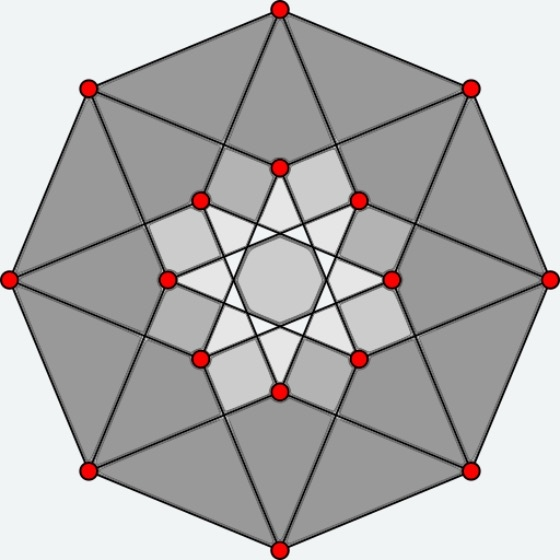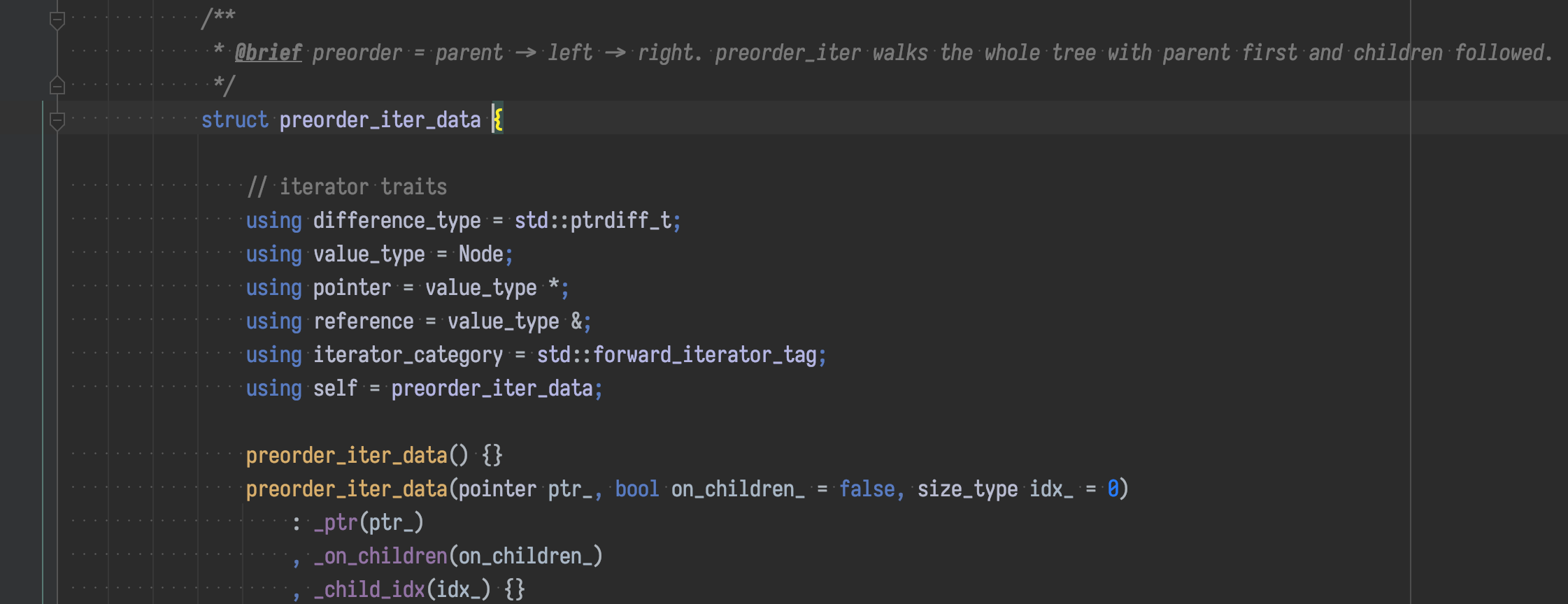# 浅谈如何实现自定义的 iterator 之二hedzr## 实现你自己的迭代器 II

### tree_t 的实现

#### tree_t

namespace dp::tree{  template<typename Data, typename Node = detail::generic_node_t<Data>>  class tree_t : detail::generic_tree_ops<Node> {    public:    using Self = tree_t<Data, Node>;    using BaseT = detail::generic_tree_ops<Node>;    using NodeT = Node;    using NodePtr = Node *;    using iterator = typename Node::iterator;    using const_iterator = typename Node::const_iterator;    using reverse_iterator = typename Node::reverse_iterator;    using const_reverse_iterator = typename Node::const_reverse_iterator;    using difference_type = std::ptrdiff_t;    using value_type = typename iterator::value_type;    using pointer = typename iterator::pointer;    using reference = typename iterator::reference;    using const_pointer = typename iterator::const_pointer;    using const_reference = typename iterator::const_reference;    ~tree_t() { clear(); }    void clear() override {      if (_root) delete _root;      BaseT::clear();    }    void insert(Data const &data) {      if (!_root) {        _root = new NodeT{data};        return;      }      _root->insert(data);    }    void insert(Data &&data) {      if (!_root) {        _root = new NodeT{data};        return;      }      _root->insert(std::move(data));    }    template<typename... Args>    void emplace(Args &&...args) {      if (!_root) {        _root = new NodeT{std::forward<Args>(args)...};        return;      }      _root->emplace(std::forward<Args>(args)...);    }    Node const &root() const { return *_root; }    Node &root() { return *_root; }    iterator begin() { return _root->begin(); }    iterator end() { return _root->end(); }    const_iterator begin() const { return _root->begin(); }    const_iterator end() const { return _root->end(); }    reverse_iterator rbegin() { return _root->rbegin(); }    reverse_iterator rend() { return _root->rend(); }    const_reverse_iterator rbegin() const { return _root->rbegin(); }    const_reverse_iterator rend() const { return _root->rend(); }    private:    NodePtr _root{nullptr};  }; // class tree_t} // namespace dp::tree

#### generic_node_t

namespace dp::tree::detail{  template<typename Data>  struct generic_node_t {    using Node = generic_node_t<Data>;    using NodePtr = Node *; //std::unique_ptr<Node>;    using Nodes = std::vector<NodePtr>;    private:    Data _data{};    NodePtr _parent{nullptr};    Nodes _children{};        // ...  }}

#### 正向迭代器

namespace dp::tree::detail{  template<typename Data>  struct generic_node_t {    // ...    struct preorder_iter_data {      // iterator traits      using difference_type = std::ptrdiff_t;      using value_type = Node;      using pointer = value_type *;      using reference = value_type &;      using iterator_category = std::forward_iterator_tag;      using self = preorder_iter_data;      using const_pointer = value_type const *;      using const_reference = value_type const &;      preorder_iter_data() {}      preorder_iter_data(pointer ptr_, bool invalid_ = false)        : _ptr(ptr_)          , _invalid(invalid_) {}      preorder_iter_data(const preorder_iter_data &o)        : _ptr(o._ptr)          , _invalid(o._invalid) {}      preorder_iter_data &operator=(const preorder_iter_data &o) {        _ptr = o._ptr, _invalid = o._invalid;        return *this;      }      bool operator==(self const &r) const { return _ptr == r._ptr && _invalid == r._invalid; }      bool operator!=(self const &r) const { return _ptr != r._ptr || _invalid != r._invalid; }      reference data() { return *_ptr; }      const_reference data() const { return *_ptr; }      reference operator*() { return data(); }      const_reference operator*() const { return data(); }      pointer operator->() { return &(data()); }      const_pointer operator->() const { return &(data()); }      self &operator++() { return _incr(); }      self operator++(int) {        self copy{_ptr, _invalid};        ++(*this);        return copy;      }      static self begin(const_pointer root_) {        return self{const_cast<pointer>(root_)};      }      static self end(const_pointer root_) {        if (root_ == nullptr) return self{const_cast<pointer>(root_)};        pointer p = const_cast<pointer>(root_), last{nullptr};        while (p) {          last = p;          if (p->empty())            break;          p = &((*p)[p->size() - 1]);        }        auto it = self{last, true};        ++it;        return it;      }      private:      self &_incr() {        if (_invalid) {          return (*this);        }        auto *cc = _ptr;        if (cc->empty()) {          Node *pp = cc;          size_type idx;          go_up_level:          pp = pp->parent();          idx = 0;          for (auto *vv : pp->_children) {            ++idx;            if (vv == _ptr) break;          }          if (idx < pp->size()) {            _ptr = &((*pp)[idx]);          } else {            if (pp->parent()) {              goto go_up_level;            }            _invalid = true;          }        } else {          _ptr = &((*cc));        }        return (*this);      }      pointer _ptr{};      bool _invalid{};      // size_type _child_idx{};    };    using iterator = preorder_iter_data;    using const_iterator = iterator;    iterator begin() { return iterator::begin(this); }    const_iterator begin() const { return const_iterator::begin(this); }    iterator end() { return iterator::end(this); }    const_iterator end() const { return const_iterator::end(this); }    // ...  }}

#### 需要照顾到的事情

1. begin() 和 end()

2. 迭代器嵌入类（不必被限定为嵌入），至少实现：

3. 递增运算符重载，以便行走

4. 递减运算符重载，如果是双向行走（bidirectional_iterator_tag）或随机行走（random_access_iterator_tag）

5. operator* 运算符重载，以便迭代器求值：使能 (*it).xxx

6. 配套实现 operator-> ，以使能 it->xxx

7. operator!= 运算符重载，以便计算迭代范围；必要时也可以显式重载 operator==（默认时编译器自动从 != 运算符上生成一个配套替代品）

1. 为了能与 STL 的 <algorithm> 算法兼容，你需要手动定义 iterator traits，如同这样：

   struct preorder_iter_data {        // iterator traits     using difference_type = std::ptrdiff_t;     using value_type = Node;     using pointer = value_type *;     using reference = value_type &;     using iterator_category = std::forward_iterator_tag;   }

1. 绝大多数情况下，你声明 std::forward_iterator_tag 类型，并定义 ++ 运算符与其配套；如果你定义为 std::bidirectional_iterator_tag 类型，那么还需要定义 -- 运算符。

2. 自增自减运算符需要同时定义前缀与后缀，请参考上一篇文章 浅谈如何实现自定义的 iterator 中的有关章节。

3. 在迭代器中，定义 begin() 与 end()，以便在容器类中借用它们（在本文的 tree_t 示例中，容器类指的是 generic_node_t。

4. 如果你想要定义 rbegin/rend，它们并不是 -- 的替代品，它们通常需要你完全独立于正向迭代器而单独定义另外一套。在 tree_t 中对此有明确的实现，但本文中限于篇幅不予列出，如果你感兴趣的话，请查阅源代码 dp-tree.hhtree.cc

### 使用/测试代码

void test_g_tree() {  dp::tree::tree_t<tree_data> t;  UNUSED(t);  assert(t.rbegin() == t.rend());  assert(t.begin() == t.end());  std::array<char, 128> buf;  //     1  // 2 3 4 5 6 7  for (auto v : {1, 2, 3, 4, 5, 6, 7}) {    std::sprintf(buf.data(), "str#%d", v);    // t.insert(tree_data{v, buf.data()});    tree_data vd{v, buf.data()};    t.insert(std::move(vd));    // tree_info(t);  }  {    auto v = 8;    std::sprintf(buf.data(), "str#%d", v);    tree_data td{v, buf.data()};    t.insert(td);    v = 9;    std::sprintf(buf.data(), "str#%d", v);    t.emplace(v, buf.data());    {      auto b = t.root().begin(), e = t.root().end();      auto &bNode = (*b), &eNode = (*e);      std::cout << "::: " << (*bNode) << '\n'; // print bNode.data()      std::cout << "::: " << (eNode.data()) << '\n';    }    {      int i;      i = 0;      for (auto &vv : t) {        std::cout << i << ": " << (*vv) << ", " << '\n';        if (i == 8) {          std::cout << ' ';        }        i++;      }      std::cout << '\n';    }    using T = decltype(t);    auto it = std::find_if(t.root().begin(), t.root().end(), [](typename T::NodeT &n) -> bool { return (*n) == 9; });    v = 10;    std::sprintf(buf.data(), "str#%d", v);    it->emplace(v, buf.data());    v = 11;    std::sprintf(buf.data(), "str#%d", v);    (*it).emplace(v, buf.data());    #if defined(_DEBUG)    auto const itv = t.find([](T::const_reference n) { return (*n) == 10; });    assert(*(*itv) == 10);    #endif  }  //  int i;  i = 0;  for (auto &v : t) {    std::cout << i << ": " << (*v) << ", " << '\n';    if (i == 8) {      std::cout << ' ';    }    i++;  }  std::cout << '\n';  i = 0;  for (auto it = t.rbegin(); it != t.rend(); ++it, ++i) {    auto &v = (*it);    std::cout << i << ": " << (*v) << ", " << '\n';    if (i == 8) {      std::cout << ' ';    }  }  std::cout << '\n';}

## 后记

:end:### hedzr

https://hedzr.com

## 评论